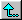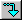Basic -- Zero Line ==> Zero Box -- EasyBasic -- Zero Line ==> Zero Box   Easy   ID=7070   Print   (submitted by Calvin Campbell)Complete Record
(Zero Line)
1/2 Square Thru,
Step To An Ocean Wave,
Men Circulate,
Right & Left Thru
(Zero Box)

Basic -- Zero Line ==> Zero Box   Easy   ID=7063   Print   (submitted by Calvin Campbell)Complete Record
(Zero Line)
Star Thru,
Dive Thru,
Centers Pass Thru,
Step To An Ocean Wave,
Men Circulate,
Right & Left Thru
(Zero Box)

Basic -- Zero Line ==> Zero Box   Easy   ID=7085   Print   (submitted by Calvin Campbell)Complete Record
(Zero Line)
Pass Thru,
Wheel & Deal,
Centers Right & Left Thru,
Centers Pass Thru
(Zero Box)

Basic -- Zero Line ==> Zero Box   Easy   ID=6404   Print   (submitted by Kazy Suzuki)Complete Record
(Zero Line)
Right & Left Thru,
Pass The Ocean,
Girls Circulate (in Wave)
(Zero Box)

 Basic -- Zero Line ==> Zero Box -- MediumBasic -- Zero Line ==> Zero Box   Medium   ID=5827   PrintComplete Record
(Zero Line)
1/2 Square Thru,
Boys Circulate,
Right & Left Thru
(Zero Box)

Basic -- Zero Line ==> Zero Box   Medium   ID=5826   PrintComplete Record
(Zero Line)
Pass The Ocean,
Swing Thru,
Ends Circulate,
Boys Run,
Wheel & Deal,
Right & Left Thru
(Zero Box)

Basic -- Zero Line ==> Zero Box   Medium   ID=7054   Print   (submitted by Calvin Campbell)Complete Record
(Zero Line)
Right & Left Thru,
Pass The Ocean,
(Zero Box)

Ends in a Wave

 Mainstream -- Zero Line ==> Zero Box -- EasyMainstream -- Zero Line ==> Zero Box   Easy   ID=7124   Print   (submitted by Calvin Campbell)Complete Record
(Zero Line)
Pass The Ocean,
Swing Thru,
Men Cross Run,
Left Swing Thru,
Men Cross Run,
Right & Left Thru,
Eight Chain 2
(Zero Box)

 Mainstream -- Zero Line ==> Zero Box -- MediumMainstream -- Zero Line ==> Zero Box   Medium   ID=6010   PrintComplete Record
(Zero Line)
Right & Left Thru,
Spin The Top,
Boys Run,
Bend The Line,
Slide Thru
(Zero Box)

 Plus -- Zero Line ==> Zero Box -- EasyPlus -- Zero Line ==> Zero Box   Easy   ID=6316   Print   (submitted by Vic Ceder)Complete Record
(Zero Line)
Touch 1/4,
Coordinate,
Bend The Line,
Right & Left Thru,
(Zero Box)

Plus -- Zero Line ==> Zero Box   Easy   ID=6160   PrintComplete Record
(Zero Line)
Touch 1/4,
Coordinate,
Bend The Line,
Right & Left Thru,
Slide Thru
(Zero Box)

Plus -- Zero Line ==> Zero Box   Easy   ID=6314   Print   (submitted by Vic Ceder)Complete Record
(Zero Line)
Right & Left Thru,
Spin Chain & Exchange The Gears,
Recycle
(Zero Box)

Plus -- Zero Line ==> Zero Box   Easy   ID=6317   Print   (submitted by Vic Ceder)Complete Record
(Zero Line)
Right & Left Thru,
Pass The Ocean,
Grand Swing Thru,
Boys Run,
Bend The Line
(Zero Box)

Plus -- Zero Line ==> Zero Box   Easy   ID=6323   Print   (submitted by Vic Ceder)Complete Record
(Zero Line)
Right & Left Thru,
Pass Thru,
3/4 Tag,
Centers Swing Thru,
Others U-Turn Back,
Extend,
Swing Thru,
(Zero Box)

Plus -- Zero Line ==> Zero Box   Easy   ID=8854   Print   (submitted by Vic Ceder)Complete Record
(Zero Line)
Right & Left Thru,
Pass The Ocean,
Girls Circulate Twice,
Spin Chain & Exchange The Gears,
Girls Circulate Twice,
Recycle
(Zero Box)

 Plus -- Zero Line ==> Zero Box -- MediumPlus -- Zero Line ==> Zero Box   Medium   ID=6327   Print   (submitted by Vic Ceder)Complete Record
(Zero Line)
Right & Left Thru,
Dixie Style To A Wave,
Boys Cast Off 3/4, Girls U-Turn Back,
Diamond Circulate,
Girls Swing Thru,
Flip The Diamond,
Spin The Top,
Linear Cycle
(Zero Box)

Plus -- Zero Line ==> Zero Box   Medium   ID=6332   Print   (submitted by Vic Ceder)Complete Record
(Zero Line)
Right & Left Thru,
Pass The Ocean,
Acey Deucey,
Boys Run,
Ferris Wheel,
Double Pass Thru,
Track 2,
Recycle
(Zero Box)

 A1 -- Zero Line ==> Zero Box -- EasyA1 -- Zero Line ==> Zero Box   Easy   ID=1144   Print   (submitted by Lloyd Lewis)Complete Record
(Zero Line)
Right And Left Thru,
Pass Thru,
Wheel And Deal,
Zoom,
Centers Swap Around
(Zero Box)

 A2 -- Zero Line ==> Zero Box -- EasyA2 -- Zero Line ==> Zero Box   Easy   ID=1326   Print   (submitted by Lloyd Lewis)Complete Record
(Zero Line)
Right And Left Thru,
Left Wheel Thru,
Clover And (Centers) Wheel Thru,
Pass & Roll,
Slip,
Centers Run,
Those Facing Out Wheel And Deal,
Others Turn & Deal
(Zero Box)

https://www.ceder.net/choreodb/view.php?action=query&DesiredSeqType=110&DesiredLevel=10&DesiredHandle=865
03-August-2020 10:31:43